Next: Order vs. Algebraic Topology Up: Reduction Theorems Previous: Fixed Point Theorems/Reflection Conditions

## Isotone Relations

Isotone relations are a beautiful variation on the order-preserving mapping theme that has a nice solution to its analogue of Problem 1.1 in the finite case. Most results in this section are due to or inspired by Walker (cf. ). For more on the relational fixed point property consider , , , .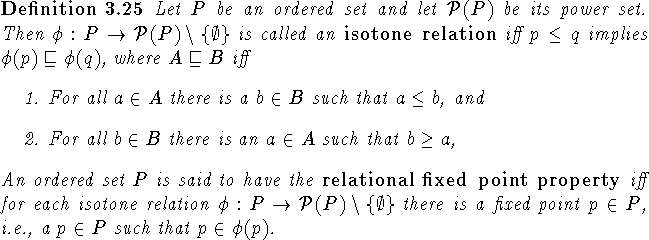Clearly the relational fixed point property implies the fixed point property and it is also preserved by retractions. Unfortunately as pointed out in , p.28, (2) or , bottom of p.161, the set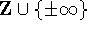with its natural order does not have the relational fixed point property. Thus the restriction to ordered sets with no infinite chains is only natural. In ordered sets with no infinite chains it is then easy to prove (cf. , Proposition 5.2) that if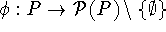is an isotone relation and there are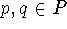such that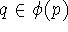and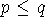, then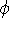has a fixed point. This allows us to reflect the relational fixed point property: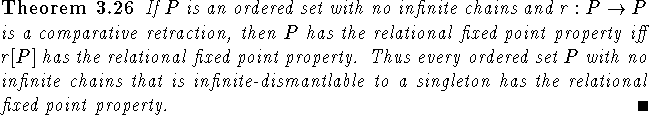Since (cf. , Theorem 5.6) an ordered set P with no infinite chains, the relational fixed point property and more than one point must have an irreducible point we arrive at the conclusion that the situation described above might be the only case that occurs. To back this conjecture we present: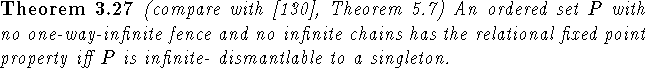Proof: By the main result in , the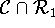-core of P exists and by Theorem 5.6 in  it has the relational fixed point property iff it is a singleton. \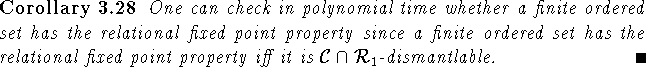We can also use these results to obtain a quick proof of the finite case of Fofanova and Rutkowski's result on ordered sets of width 2 (recall that the width of an ordered set P is the size of the largest antichain in P, where an antichain is a subset of pairwise noncomparable elements):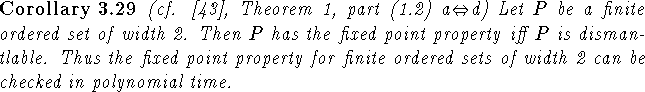Proof: We will show that P has the fixed point property iff P has the relational fixed point property, which by Theorem 3.27 implies the result. It is clear that the relational fixed point property implies the fixed point property. Now suppose P does not have the relational fixed point property. Letbe an isotone relation with no fixed point. Since P has width 2,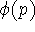is a chain for each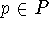. Now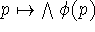is a fixed point free order-preserving map. \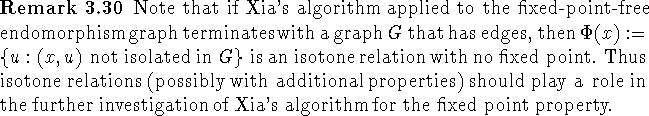Next: Order vs. Algebraic Topology Up: Reduction Theorems Previous: Fixed Point Theorems/Reflection Conditions

Bernd.S.W.Schroder